##### How to Use array_dims() Function in PostgreSQL

PostgreSQL offers a wide range of array functions, such as ARRAY_CAT(), ARRAY_REPLACE(), ARRAY_APPEND(), and so on. These functions can manipulate the array or get some information about the arrays depending upon how the function works. The array_dims() is one of the array functions that is used to find out the dimensions of an array.

Let's see how we use the array_dims() function.

How to Use array_dims() Function in PostgreSQL?

The array_dims() gives out the information about the dimensions of an array. The array_dims() takes an array as a parameter and returns text. The basic syntax for the function is given below:

`array_dims(Array) -> TEXT`

The array whose dimensions are to be checked is passed as an argument in the function. If the value in the function is NULL it will throw an error. The function returns a text having information about the dimensions of the array.

Let’s understand the topic better with an example.

Example

Let's consider an array; [‘John’, ’Peter’]. If we want to get information about this particular array we will use the array_dims() function as follows:

`SELECT array_dims(ARRAY['John','Peter']);`

When we execute the query, it will give the information about the dimensions of this array: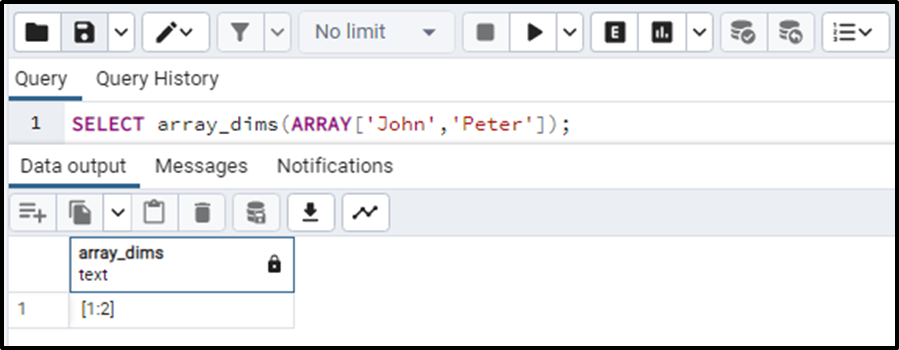So the above query has returned “[1,2]”, which simply means the array passed is a one-dimensional array having indexes starting from 1 to 2.

Consider another example so that it is more clear to us:

```SELECT array_dims(ARRAY[
['John','Peter'],
['Willams','Sarah'],
['katherine','Alex']
]);```

The output of the given query gives: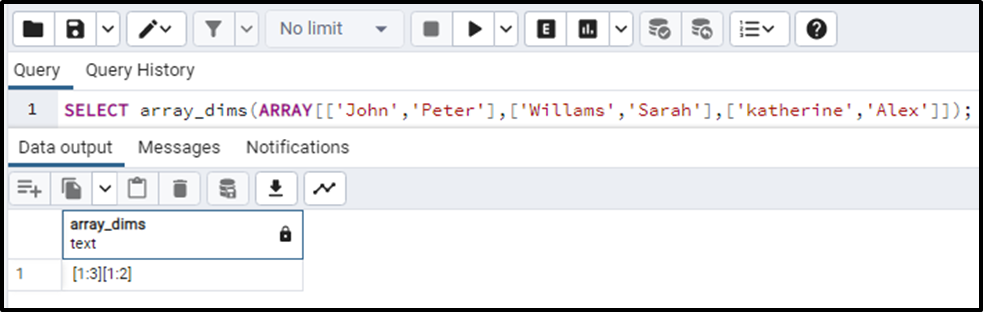The two arrays for dimensions represent that the array is a 2-dimensional array, where the first array starts from index 1 to 3 and the second-dimensional array starts from index 1 to index 2.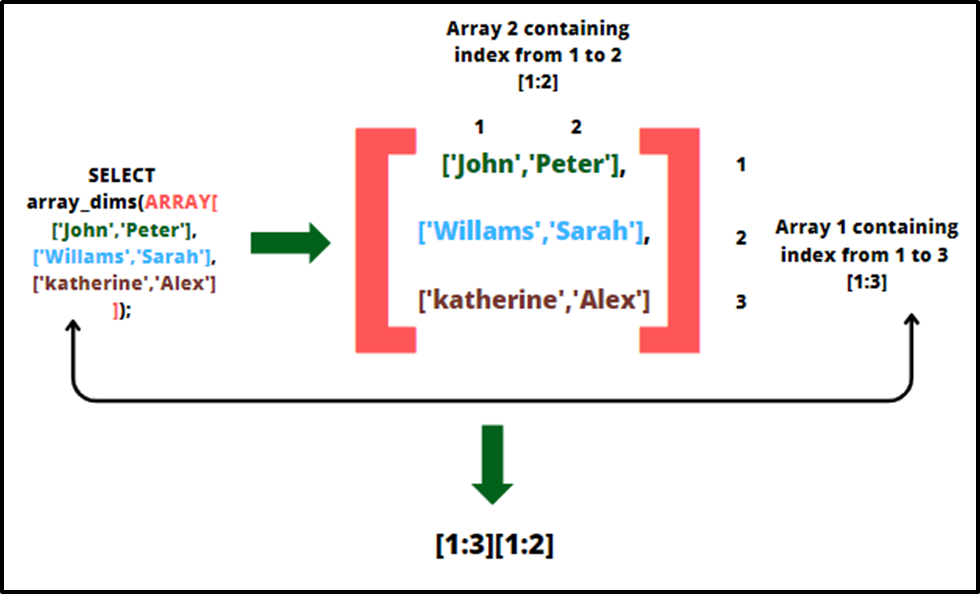Consider another example with a slightly more complexity level to check our concepts:

```SELECT array_dims(ARRAY[
[['John','Peter']],
[['Willams','Sarah']],
[['katherine','Alex']]
]);```

The output of the example is: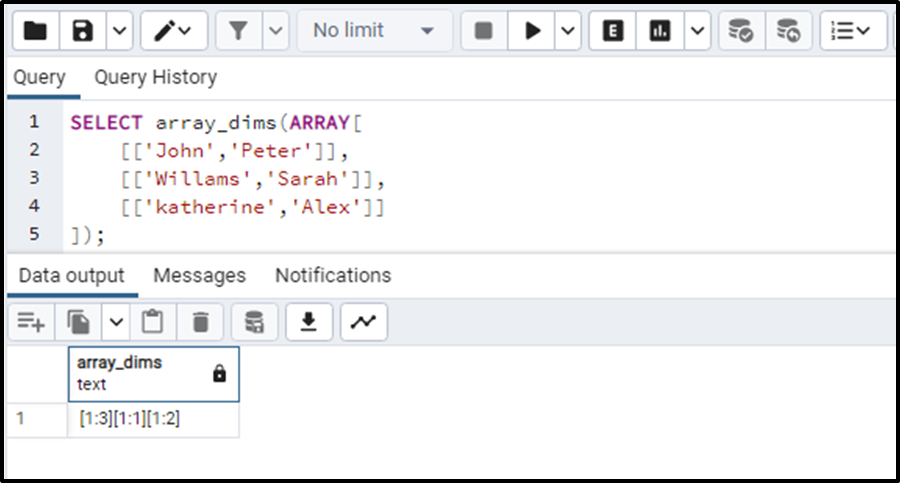This clearly means that the array is 3-dimensional. The first-dimensional array starts from index 1 to 3, the second-dimensional array has index 1 to 1 means only one array in it, and the three-dimensional array has indexes from 1 to 3.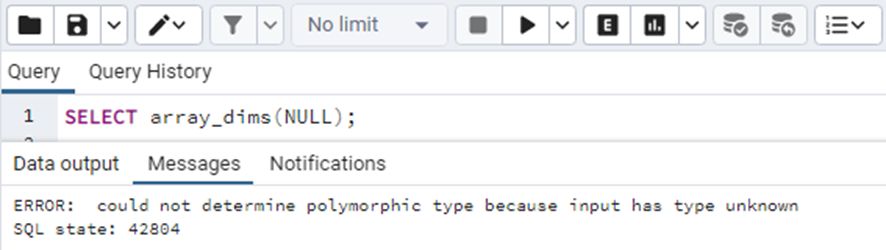If we pass the array argument as NULL it will always throw an error.

Conclusion

In order to get the dimension of a specific array we use the array_dims() function. An input array is provided to the function as a parameter and returns the information about its dimensions. If the argument in the function is NULL it will return an error. This post covered the functioning of the array_dims() function with the help of some practical examples.